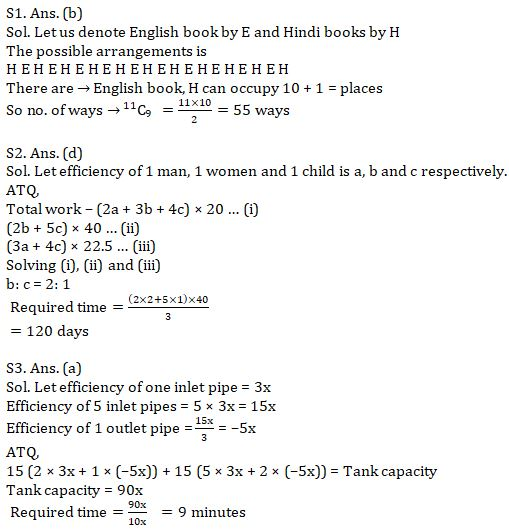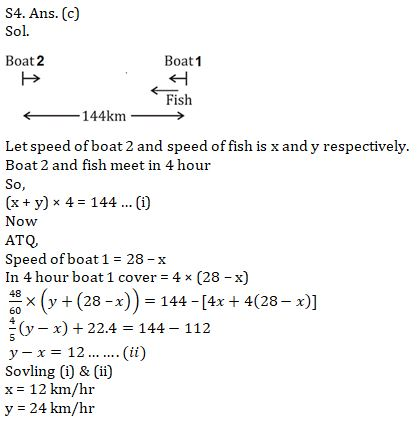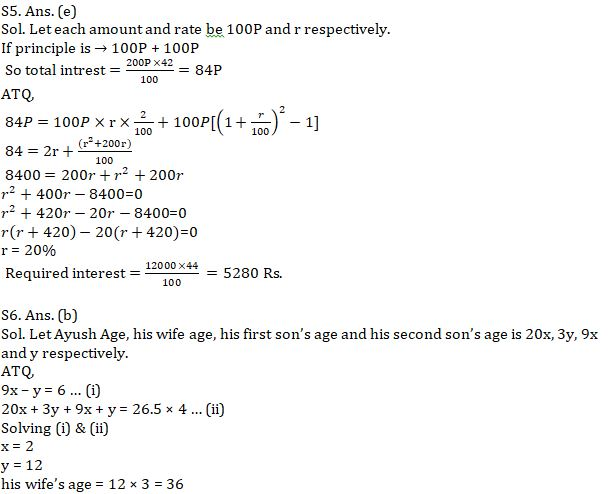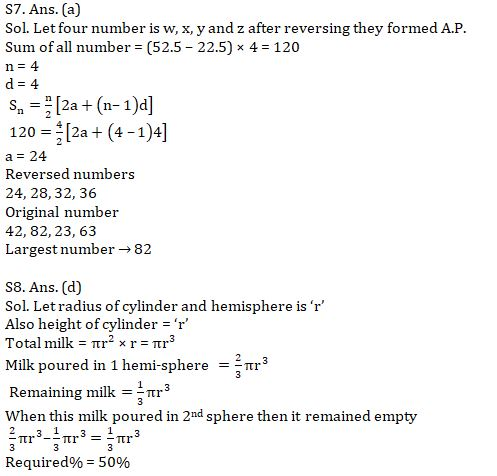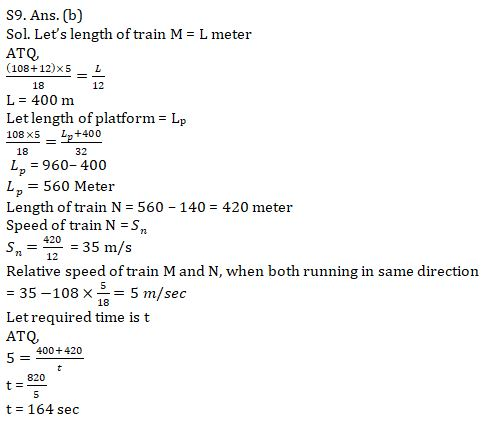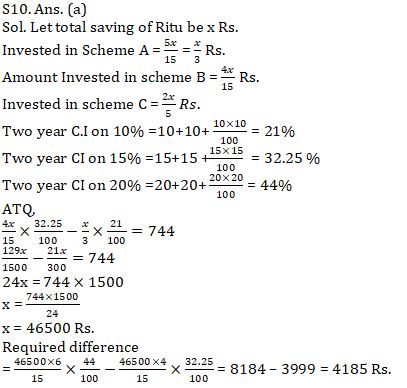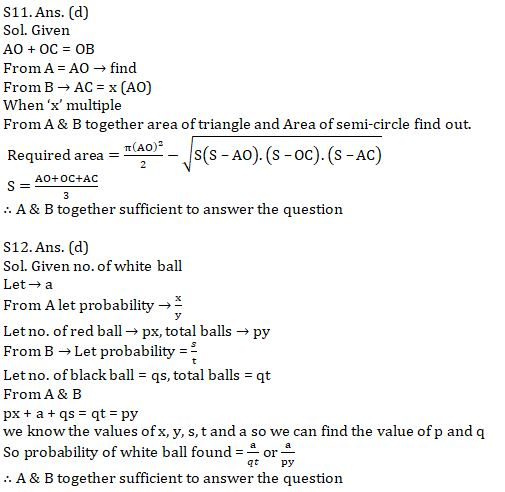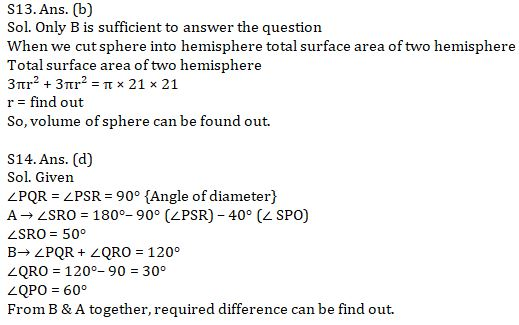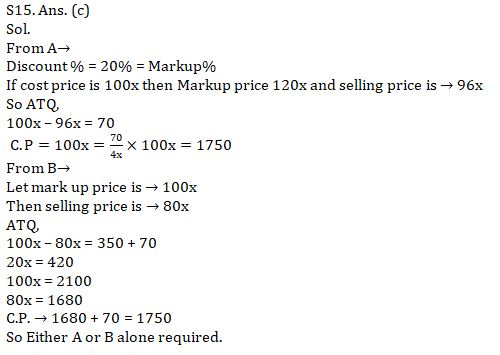Latest Banking jobs   »   Quantitative Aptitude Quiz For SBI Clerk...

# Quantitative Aptitude Quiz For SBI Clerk Mains Exam 2021- 24th August

Q1. In how many ways 9 hindi books are placed between 10 English books such that no two Hindi book comes together?
(a) 60
(b) 55
(c) 45
(d) 96
(e) 75

Q2. 2 men, 3 women and 4 children can do work in 10 days. 2 women and 5 children can do the same work in 40 days. Find the time taken by 1 women and 1 child to do the same work if 3 man and 4 children do the same work in 22.5 days.
(a) 96 days
(b) 124 days
(c) 144 days
(d) 120 days
(e) 90 days

Q3. 5 inlet pipes (same capacity) can fill a tank in same time in which 3 outlet pipes (same capacity) can empty it. If 2 inlet and one outlet pipe is opened for first minutes and 5 inlet and 2 outlet pipe open for 2nd minute and process continues till tank is completely filled in 30 minutes. Find the time in which 2 outlet pipe can empty the completely filled tank.
(a) 9 minute
(b) 12 minutes
(c) 6 minutes
(d) 10 minutes
(e) 15 minutes

Q4. A lake in which speed of water current is zero has a stretch of 144 km. Two boats, whose sum of speed in still water is 28 km/hr, starts from two ends of lake. A fish starts swimming simultaneously with one boat toward another boat and reach the second boat in 4 hours and then returns toward first boat and touch it in 48 minutes. Find the speed of fish.
(a) 12 km/hr
(b) 16 km/hr
(c) 24 km/hr
(d) 40 km/hr
(e) 8 km/hr

Q5. Two equal amounts invested into two schemes for two year which offer S.I. & C.I. respectively for same rate. Total profit earned after two years is 42%. If 12000 Rs. invested with same rate for 2 years on C.I., Find interest earned?
(a) 5680 Rs.
(b) 4720 Rs.
(c) 4680 Rs.
(d) 4350 Rs.
(e) 5280 Rs.

Q6. Ratio of Ayush Age to his first son’s age is 20 : 9 and Ayush wife’s age to Ayush 2nd son’s age is 3 : 1. If elder son of Ayush is 6 year elder than another son (ayush has only two son and no daughter) and average age of family is 26.5 year then find the age of Ayush’s wife.
(a) 24
(b) 36
(c) 40
(d) 38
(e) 28

Q7. Ritu take four, two-digit numbers and take average of these number which is 52.5. If she reversed all the digits of 4 numbers she found that their average is now 22.5 less than the previous one and they formed A.P having common difference 4. Find the largest number.
(a) 82
(b) 80
(c) 36
(d) cannot be determined
(e) 64

Q8. A cylinder whose height is equal to its radius is full of milk. Its milk is poured into a hemi spherical bowl of same radius as of cylinder and remaining milk is poured into another hemi-spherical bowl of same volume as of previous one. What percentage of the volume of second hemispherical bowl remained empty?
(a) 40%
(b) 66⅔%
(c) 33⅓%
(d) 50%
(e) 100%

Q9. A train M running at the speed of 108 km/hr crosses a man running at 12 km/hr in opposite direction of train in 12 second and crosses a platform in 32 sec. If a train N is Standing on same platform and length of platform is 140 meter more than length of train N. Find in what time train N will cross train M running in same direction, if train N passes a pole in 12 second?
(a) 168 sec
(b) 164 sec
(c) 154 sec
(d) 186 sec
(e) 172 sec

Q10. Ritu invested her total saving in three different FD schemes A, B and C in the ratio of 5 : 4 : 6 on CI for two years at the rate of 10%, 15% and 20% respectively.If interest is calculated annually and interest from scheme B is Rs. 744 more than interest from scheme A then, find difference between interest received from scheme C and scheme B by Ritu?
(a) Rs. 4185
(b) Rs. 4175
(c) Rs. 3840
(d) Rs. 4580
(e) Rs. 3250

Directions(11-15): The following questions are accompanied by two statements (A) and (B) . You have to determine which statements(s) is/are sufficient/necessary to answer the questions.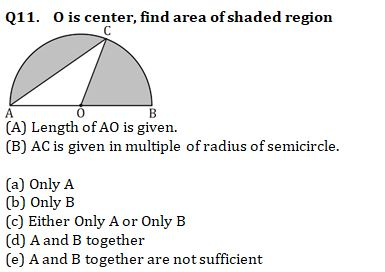Q12. In a box three type of balls are there, Black, Red and White. If no. of white balls is given then find out the probability of getting one white ball.
(A) Probability of getting one Red ball is given.
(B) Probability of getting one black ball is given.

(a) Only A
(b) Only B
(c) Either Only A or Only B
(d) A and B together
(e) A and B together are not sufficient

Q13. What is the volume of the sphere?
(A) Surface area of hemisphere is equal to the total surface area of the cylinder having radius and height in ratio 3 : 4.
(B) When we cut sphere into two hemi-sphere then total surface area is equal to the area of a circle whose radius is 21 cm.

(a) Only A
(b) Only B
(c) Either Only A or Only B
(d) A and B together
(e) A and B together are not sufficient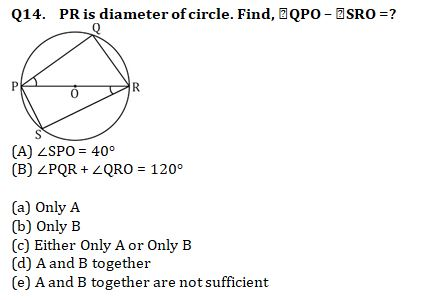Q15. A shopkeeper gets a loss of 70 Rs. when he sold an article at 20% discount on M.P. Find cost price of Article.
(A) % of mark up above cost price is equal to % discount given on M.P.
(B) when no discount is given, article sold at profit of 350 Rs.

(a) Only A
(b) Only B
(c) Either Only A or Only B
(d) A and B together
(e) A and B together are not sufficient

Solutions: